# Precalculus : Fundamental Theorem of Algebra

## Example Questions

1 3 Next →

### Example Question #9 : Find Complex Zeros Of A Polynomial Using The Fundamental Theorem Of Algebra

Find all the real and complex zeros of the following equation: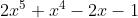Possible Answers: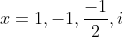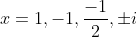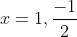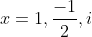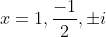Correct answer:Explanation:

First, factorize the equation using grouping of common terms: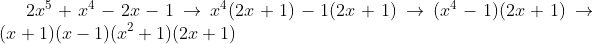Next, setting each expression in parentheses equal to zero yields the answers.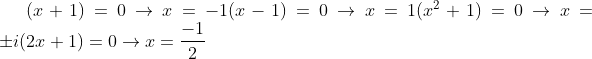### Example Question #10 : Find Complex Zeros Of A Polynomial Using The Fundamental Theorem Of Algebra

Find all the zeroes of the following equation and their multiplicity: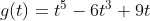Possible Answers: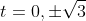(multiplicity of 2 on 0, multiplicity of 1 on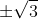)(multiplicity of 1 on 0, multiplicity of 2 on)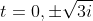(multiplicity of 1 on 0, multiplicity of 2 on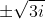)(multiplicity of 2 on 0, multiplicity of 1 on)

Correct answer:(multiplicity of 1 on 0, multiplicity of 2 on)

Explanation:

First, pull out the common t and then factorize using quadratic factoring rules: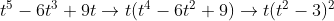This equation has a solution as two values: when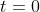, and when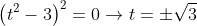. Therefore, But since the degree on the former equation is one and the degree on the latter equation is two, the multiplicities are 1 and 2 respectively.

### Example Question #21 : Fundamental Theorem Of Algebra

Find a fourth-degree polynomial whose zeroes are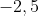, and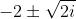Possible Answers: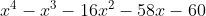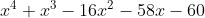Correct answer:Explanation:

This one is a bit of a journey. The expressions for the first two zeroes are easily calculated,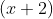and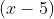respectively. The last expression must be broken up into two equations: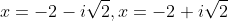which are then set equal to zero to yield the expressions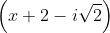andFinally, we multiply together all of the parenthesized expressions, which multiplies out to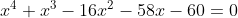### Example Question #22 : Fundamental Theorem Of Algebra

The third-degree polynomial expression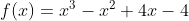has a real zero at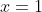. Find all of the complex zeroes.

Possible Answers:
Explanation:

First, factor the expression by grouping: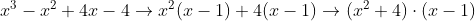To find the complex zeroes, set the term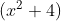equal to zero: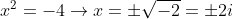1 3 Next →

### All Precalculus Resources